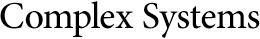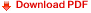## Complexity Growth in Almost Periodic Fluids in the Case of Lattice Gas Cellular Automata and Vlasov SystemsO. Knill
Department of Mathematics,
University of Arizona,
Tucson, AZ, 85721, USA

E. Reed
Department of Applied Physics,
Caltech,
Pasadena, CA, 91125, USA

#### Abstract

Two examples of dynamical systems with spatial almost periodicity are considered and the complexity growth during the time evolution is quantified.

The first example deals with almost periodic cellular automata (CA). The growth rate of the information which is needed to store a configuration can quantitatively be given by a real number which is bounded above by the dimension of the CA.

In the second example, where periodicity is understood in the classic sense of Bohr, the collision-free Boltzmann equation is considered. The method of characteristics allows the proof of an existence theorem for almost periodic initial conditions. A Lyapunov exponent measures the growth of complexity during the evolution.